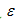### Introduction

There are different loading configurations of a cantilever beam, such as end load, end moment, uniformly load distribution, intermediate load, and triangular load distribution. The idea is that due to these loading configuration how the moment and stress changes along the surface of a cantilever beam. Since going through all of these situations is a long procedure, an end-load configuration of a cantilever beam with a rectangular cross-section is chosen to describe the variance of moment and stress along the surface and write equations of moment and stress in this situation.
As has been shown in Figure 1, one end of the beam is cantilevered and clamped to a support and a load P is applied at the free end of the beam. A strain gage is also mounted on the surface of the beam at a distance x from the clamped end of the beam. From this geometry, we can obtain equations for bending moment and stress at gage location.
where M is the bending moment at gage location, P is applied load at free end of the beam, L is distance of load application, and x is distance of gage location from the clamped end of the beam. The stress on the surface of the beam at gage location can be calculated from the following equation:

where: is bending stress at the surface of the beam at a distance L-x from the point of load application,  M(x) is bending moment at distance x, c is the distance from natural axis of the beam to the beam’s surface, which is t/2, and I=bt3/12 is moment of inertia of the beam’s cross-section.

By combing equations (1) and (2), we can obtain stress as function of load and geometry.
From Hook’s Law, we can show the relationship between stress and strain along the beam’s surface.
where E is Young’s Modulus.
Sine the relationship between load, stress, bending moment, and the geometry have been described in equations (1) through (6), the purpose of this study was being able to calculate unknown applied weights at the free end of several cantilever beams by installing strain gages on the beams’ surfaces in a distance from beams’ clamped ends and evaluate possibility of any error during this experiment.

### Experimental Setup

Two different types of material made cantilever beams, as shown in Figure 2 , were used to perform this experiment. The first type beam was made of 2024-T4 aluminum, while the second type beam was made of 6061-T651 aluminum. The dimensions are shown in Figure 2, but the distances of load application and strain gages are different for each specimen. The varying dimensions are tabulated in Table 1. There were a total of seven specimens to be tested. Three of them were made of 2024-T4 aluminum, and four of them were made of 6061-T651 aluminum. One strain gage was mounted on each cantilever beam in a distance from the clamped end of the beam. The lead-wires of the strain gage were connected to a P-3500 strain indicator to read strain at that point.

### Experimental Procedure

The prepared cantilever beam with strain gage was clamped on the edge of a table and the lead-wires were connected to the strain indicator. An unknown load was applied on the free end of the beam. The strain at gage location was read by P-3500 strain indicator after application of the unknown load. Seven specimens were tested at same manner. The strains for all seven specimens are tabulated in Table 1.

### Data analysis

Theoretical calculation of stress and strain is presented in equations (4) and (6).  By manipulating equation (6), we are able to calculate unknown weights. Modulus of Elasticity for both types of materials are used from Module 2 (previous experiment), where the Young’s Modulus was 10.6 Msi for 6061-T651 aluminum and 9.0 Msi for 2024-T4 aluminum beams.
The unknown weights can be calculated as following:

whereis the indicated strain on the surface of beam at gage point. E is Young’s Modulus in Msi, b is beam’s width, t is beam’s thickness, L is the distance of load application on the beam, and x is the distance of gage from the clamped end. Table 1 also shows calculation of unknown weights on every beam.

### Discussion

The results show that the calculated values are very close to each others. The average weight for seven specimens are obtained 5.35 lbs. There may be some sources of errors, such as, alignment of strain gage, initial zero strain reading, and Young’s Modulus. Since experimentally mounting strain gages is a challenging procedure, it is not easy to install all gages along straight line, or strain gages may be oriented during mounting. This difficulty causes error in strain indication. Another factor is initial strain of P-3500 which might hasn’t been set to zero strain before testing. This is also a factor that causes difference in strain reading from one beam to another. The Young’s Modulus was obtained from previous experiment. Sine it wasn’t a published value for the Young’s Modulus to guarantee the accuracy, we can also take this into account for the source of error.

### Conclusion

As a conclusion, we can say that the results obtained from the experiment are reasonable. This experiment was an opportunity to get familiar with the theory of cantilever beams and being able to calculate the stresses and stains along the surface of a cantilever beam. This experiment also was a tool to obtain an unknown load at the point of application by using a strain gage on the surface of a cantilever beam at any point we want to mount.